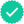#### Related questions

Question

Subject: Abstract Algebra

Suppose G is a group, with a belong to G.

a. Prove that a^ma^n = a^m+n for all natural numbers m and n. Hint: Fix n belong to N. Then prove by mathematical induction on m.

b. Prove that (a^m)^n = a^mn for all natural numbers m and n.

Expert SolutionKnowledge Booster••